Share

# NCERT solutions for Class 10 Mathematics Textbook chapter 6 - Triangles [Latest edition]

Course
Textbook page

#### Chapters## Chapter 6: Triangles

Ex. 6.1Ex. 6.2Ex. 6.3Ex. 6.4Ex. 6.5Ex. 6.6Others

#### NCERT solutions for Class 10 Mathematics Textbook Chapter 6 Triangles Exercise 6.1 [Page 122]

Ex. 6.1 | Q 1.1 | Page 122

Fill in the blanks using correct word given

All circles are __________.

• congruent

• similar

Ex. 6.1 | Q 1.2 | Page 122

Fill in the blanks using correct word given

All squares are __________.

• similar

• congruent

Ex. 6.1 | Q 1.3 | Page 122

Fill in the blanks using correct word given

All __________ triangles are similar.

• isosceles

• equilateral

Ex. 6.1 | Q 1.4 | Page 122

Fill in the blanks using correct word given in the brackets:−

Two polygons of the same number of sides are similar, if (a) their corresponding angles are __________ and (b) their corresponding sides are __________. (equal, proportional)

Ex. 6.1 | Q 2.1 | Page 122

Give two different examples of pair of similar figures

Ex. 6.1 | Q 2.1 | Page 122

Give two different examples of pair of Non-similar figures

Ex. 6.1 | Q 3 | Page 122

State whether the following quadrilaterals are similar or not: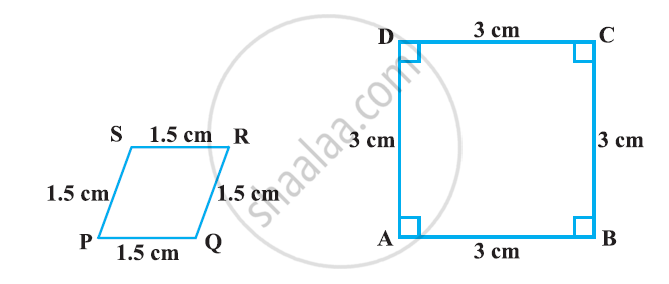#### NCERT solutions for Class 10 Mathematics Textbook Chapter 6 Triangles Exercise 6.2 [Pages 128 - 129]

Ex. 6.2 | Q 1.1 | Page 128

See the given Figure.  DE || BC. Find EC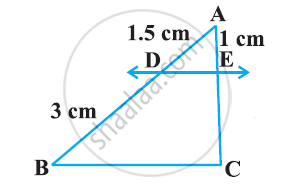Ex. 6.2 | Q 1.2 | Page 128

See the given Figure.  DE || BC. Find AD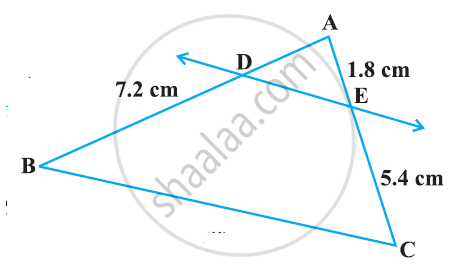Ex. 6.2 | Q 2.1 | Page 128

E and F are points on the sides PQ and PR respectively of a ΔPQR. For the following case, state whether EF || QR. PE = 3.9 cm, EQ = 3 cm, PF = 3.6 cm and FR = 2.4 cm

Ex. 6.2 | Q 2.2 | Page 128

E and F are points on the sides PQ and PR respectively of a ΔPQR. For the following case, state whether EF || QR

PE = 4 cm, QE = 4.5 cm, PF = 8 cm and RF = 9 cm

Ex. 6.2 | Q 2.3 | Page 128

E and F are points on the sides PQ and PR respectively of a ΔPQR. For the following case, state whether EF || QR. PQ = 1.28 cm, PR = 2.56 cm, PE = 0.18 cm and PF = 0.36 cm

Ex. 6.2 | Q 3 | Page 128

In the following figure, if LM || CB and LN || CD, prove that (AM)/(AB)=(AN)/(AD)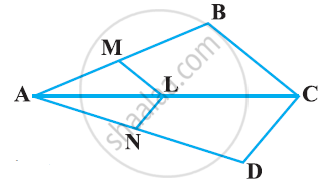Ex. 6.2 | Q 4 | Page 128

In the following figure, DE || AC and DF || AE. Prove that (BF)/(FE)=(BE)/(EC)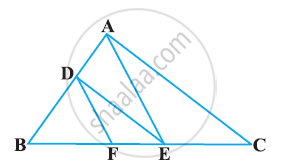Ex. 6.2 | Q 5 | Page 129

In the following figure, DE || OQ and DF || OR, show that EF || QR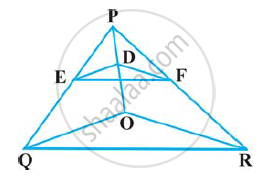Ex. 6.2 | Q 6 | Page 129

In the following figure, A, B and C are points on OP, OQ and OR respectively such that AB || PQ and AC || PR. Show that BC || QR.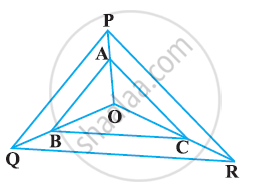Ex. 6.2 | Q 7 | Page 129

Using Basic proportionality theorem, prove that a line drawn through the mid-points of one side of a triangle parallel to another side bisects the third side. (Recall that you have proved it in Class IX).

Ex. 6.2 | Q 8 | Page 129

Using Converse of basic proportionality theorem, prove that the line joining the mid-points of any two sides of a triangle is parallel to the third side. (Recall that you have done it in Class IX).

Ex. 6.2 | Q 9 | Page 129

ABCD is a trapezium in which AB || DC and its diagonals intersect each other at the point O. Show that (AO)/(BO) = (CO)/(DO)

Ex. 6.2 | Q 10 | Page 129

The diagonals of a quadrilateral ABCD intersect each other at the point O such that (AO)/(BO) = (CO)/(DO)  Show that ABCD is a trapezium

#### NCERT solutions for Class 10 Mathematics Textbook Chapter 6 Triangles Exercise 6.3 [Pages 138 - 141]

Ex. 6.3 | Q 1.1 | Page 138

State which pair of triangles in the following figure are similar? Write the similarity criterion used by you for answering the question and also write the pairs of similar triangles in the symbolic form: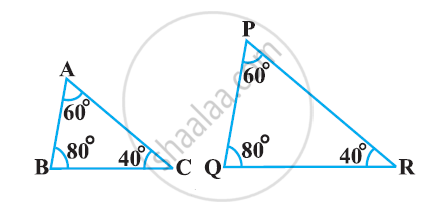Ex. 6.3 | Q 1.2 | Page 139

State which pair of triangles in the given figure are similar? Write the similarity criterion used by you for answering the question and also write the pairs of similar triangles in the symbolic form: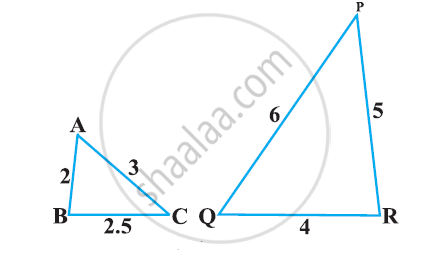Ex. 6.3 | Q 1.3 | Page 139

State which pair of triangles in the following figure are similar? Write the similarity criterion used by you for answering the question and also write the pairs of similar triangles in the symbolic form: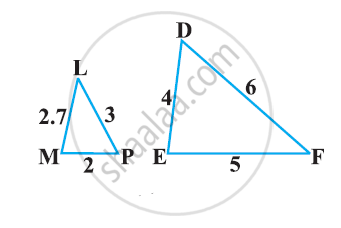Ex. 6.3 | Q 1.4 | Page 139

State which pair of triangles in the following figure are similar? Write the similarity criterion used by you for answering the question and also write the pairs of similar triangles in the symbolic form: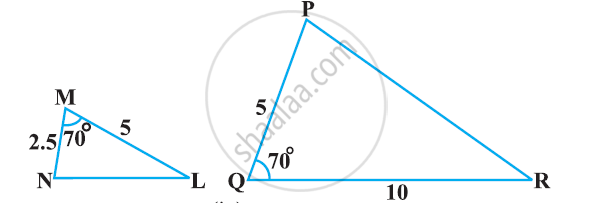Ex. 6.3 | Q 1.5 | Page 139

State which pair of triangles in the following figure are similar? Write the similarity criterion used by you for answering the question and also write the pairs of similar triangles in the symbolic form: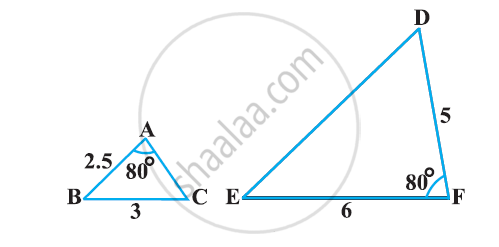Ex. 6.3 | Q 1.6 | Page 139

State which pair of triangles in the following figure are similar? Write the similarity criterion used by you for answering the question and also write the pairs of similar triangles in the symbolic form: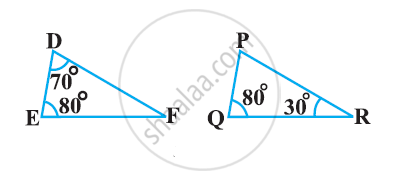Ex. 6.3 | Q 2 | Page 139

In the following figure, ΔODC ∼ ΔOBA, ∠BOC = 125° and ∠CDO = 70°. Find ∠DOC, ∠DCO and ∠OAB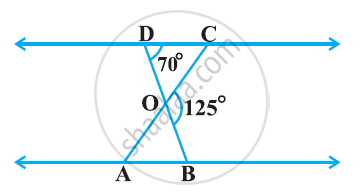Ex. 6.3 | Q 3 | Page 139

Diagonals AC and BD of a trapezium ABCD with AB || DC intersect each other at the point O. Using a similarity criterion for two triangles, show that (AO)/(OC) = (OB)/(OD)

Ex. 6.3 | Q 4 | Page 140

In the following figure, (QR)/(QS) = (QT)/(PR)  and ∠1 = ∠2. Show that ΔPQS ~ ΔTQR.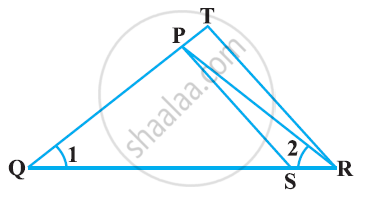Ex. 6.3 | Q 5 | Page 140

S and T are point on sides PR and QR of ΔPQR such that ∠P = ∠RTS. Show that ΔRPQ ∼ ΔRTS.

Ex. 6.3 | Q 6 | Page 140

In the following figure, if ΔABE ≅ ΔACD, show that ΔADE ∼ ΔABC.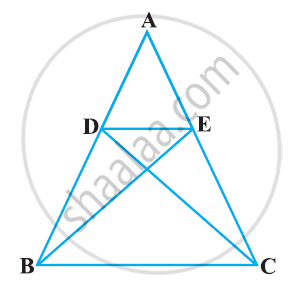Ex. 6.3 | Q 7.1 | Page 140

In the following figure, altitudes AD and CE of ΔABC intersect each other at the point P. Show that: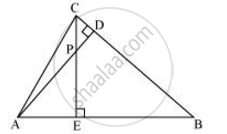ΔAEP ∼ ΔCDP

Ex. 6.3 | Q 7.2 | Page 140

In the following figure, altitudes AD and CE of ΔABC intersect each other at the point P. Show that: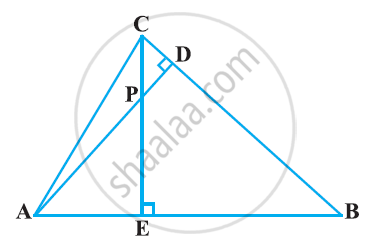ΔABD ∼ ΔCBE

Ex. 6.3 | Q 7.3 | Page 140

In the following figure, altitudes AD and CE of ΔABC intersect each other at the point P. Show that: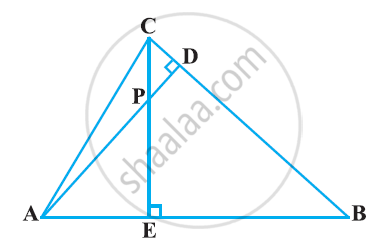Ex. 6.3 | Q 7.4 | Page 140

In the following figure, altitudes AD and CE of ΔABC intersect each other at the point P. Show that: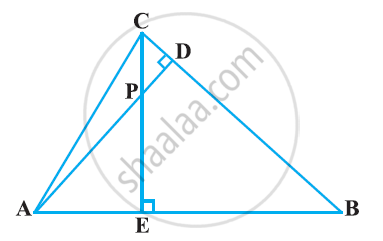ΔPDC ∼ ΔBEC

Ex. 6.3 | Q 8 | Page 140

E is a point on the side AD produced of a parallelogram ABCD and BE intersects CD at F. Show that ΔABE ∼ ΔCFB

Ex. 6.3 | Q 9 | Page 140

In the following figure, ABC and AMP are two right triangles, right angled at B and M respectively, prove that: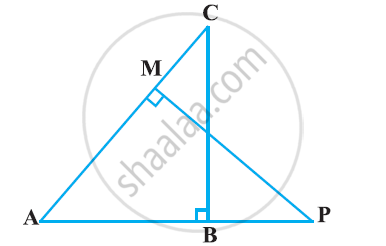ΔABC ~ ΔAMP

 (CA)/(PA) = (BC)/(MP)

Ex. 6.3 | Q 10 | Page 140

CD and GH are respectively the bisectors of ∠ACB and ∠EGF such that D and H lie on sides AB and FE of ΔABC and ΔEFG respectively. If ΔABC ~ ΔFEG, Show that

(i) (CD)/(GH) = (AC)/(FG)

(ii) ΔDCB ~ ΔHGE

(iii) ΔDCA ~ ΔHGF

Ex. 6.3 | Q 11 | Page 141

In the following figure, E is a point on side CB produced of an isosceles triangle ABC with AB = AC. If AD ⊥ BC and EF ⊥ AC, prove that ΔABD ∼ ΔECF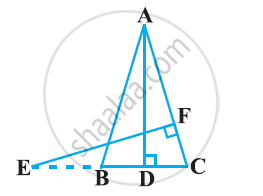Ex. 6.3 | Q 12 | Page 141

Sides AB and BC and median AD of a triangle ABC are respectively proportional to sides PQ and QR and median PM of ΔPQR (see the given figure). Show that ΔABC ∼ ΔPQR.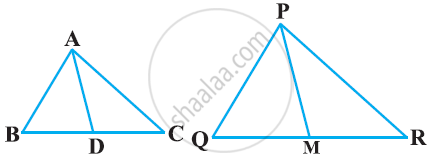Ex. 6.3 | Q 13 | Page 141

D is a point on the side BC of ∆ABC such that ∠ADC = ∠BAC. Prove that  \frac{CA}{CD}=\frac{CB}{CA} or, CA^2 = CB × CD.

Ex. 6.3 | Q 14 | Page 141

Sides AB and AC and median AD of a triangle ABC are respectively proportional to sides PQ and PR and median PM of another triangle PQR. Show that ΔABC ~ ΔPQR

Ex. 6.3 | Q 15 | Page 141

A vertical pole of a length 6 m casts a shadow 4m long on the ground and at the same time a tower casts a shadow 28 m long. Find the height of the tower.

Ex. 6.3 | Q 16 | Page 141

AD and PM are medians of triangles ABC and PQR, respectively where Δ ABC ~ Δ PQR, prove that ("AB")/("PQ") = ("AD")/("PM")

#### NCERT solutions for Class 10 Mathematics Textbook Chapter 6 Triangles Exercise 6.4 [Pages 143 - 144]

Ex. 6.4 | Q 1 | Page 143

Let Δ ABC ~ Δ DEF and their areas be, respectively, 64 cm2 and 121 cm2. If EF = 15.4 cm, find BC

Ex. 6.4 | Q 2 | Page 143

Diagonals of a trapezium ABCD with AB || DC intersect each other at the point O. If AB = 2CD, find the ratio of the areas of triangles AOB and COD.

Ex. 6.4 | Q 3 | Page 144

In Figure, ABC and DBC are two triangles on the same base BC. If AD intersects BC at O, show that (ar(ABC))/(ar(DBC)) = (AO)/(DO)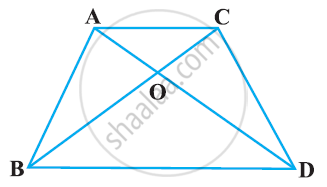Ex. 6.4 | Q 4 | Page 144

If the areas of two similar triangles are equal, prove that they are congruent

Ex. 6.4 | Q 5 | Page 144

D, E and F are respectively the mid-points of sides AB, BC and CA of ΔABC. Find the ratio of the area of ΔDEF and ΔABC.

Ex. 6.4 | Q 6 | Page 144

Prove that the ratio of the areas of two similar triangles is equal to the square of the ratio of their corresponding medians.

Ex. 6.4 | Q 7 | Page 144

Prove that the area of an equilateral triangle described on one side of a square is equal to half the area of the equilateral triangle described on one of its diagonals

Ex. 6.4 | Q 8 | Page 144

ABC and BDE are two equilateral triangles such that D is the mid-point of BC. Ratio of the area of triangles ABC and BDE is

• 2 : 1

• 1 : 2

• 4 : 1

• 1 : 4

Ex. 6.4 | Q 9 | Page 144

Sides of two similar triangles are in the ratio 4 : 9. Areas of these triangles are in the ratio

• 2 : 3

• 4 : 9

• 81 : 16

• 16 : 81

#### NCERT solutions for Class 10 Mathematics Textbook Chapter 6 Triangles Exercise 6.5 [Pages 150 - 151]

Ex. 6.5 | Q 1.1 | Page 150

Sides of triangle are given below. Determine it is a right triangle or not? In case of a right triangle, write the length of its hypotenuse. 7 cm, 24 cm, 25 cm

Ex. 6.5 | Q 1.2 | Page 150

Sides of triangles are given below. Determine it is a right triangles? In case of a right triangle, write the length of its hypotenuse. 3 cm, 8 cm, 6 cm

Ex. 6.5 | Q 1.3 | Page 150

Sides of triangle are given below. Determine it is a right triangle or not? In case of a right triangle, write the length of its hypotenuse. 50 cm, 80 cm, 100 cm

Ex. 6.5 | Q 1.4 | Page 150

Sides of triangle are given below. Determine it is a right triangle or not? In case of a right triangle, write the length of its hypotenuse. 13 cm, 12 cm, 5 cm

Ex. 6.5 | Q 2 | Page 150

PQR is a triangle right angled at P and M is a point on QR such that PM ⊥ QR. Show that PM2 = QM . MR

Ex. 6.5 | Q 3.1 | Page 150

In Figure, ABD is a triangle right angled at A and AC ⊥ BD. Show that AB2 = BC × BD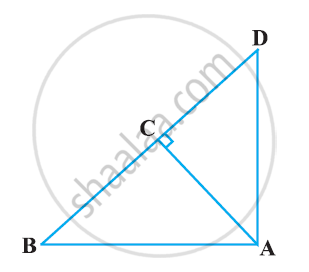Ex. 6.5 | Q 3.2 | Page 150

In Figure ABD is a triangle right angled at A and AC ⊥ BD. Show that AC2 = BC × DCEx. 6.5 | Q 3.3 | Page 150

In Figure, ABD is a triangle right angled at A and AC ⊥ BD. Show that AD2 = BD × CDEx. 6.5 | Q 4 | Page 150

ABC is an isosceles triangle right angled at C. Prove that AB2 = 2AC2

Ex. 6.5 | Q 5 | Page 150

ABC is an isosceles triangle with AC = BC. If AB2 = 2AC2, prove that ABC is a right triangle.

Ex. 6.5 | Q 6 | Page 150

ABC is an equilateral triangle of side 2a. Find each of its altitudes.

Ex. 6.5 | Q 7 | Page 150

Prove that the sum of the squares of the sides of a rhombus is equal to the sum of the squares of its diagonals

Ex. 6.5 | Q 8 | Page 151

In the following figure, O is a point in the interior of a triangle ABC, OD ⊥ BC, OE ⊥ AC and OF ⊥ AB. Show that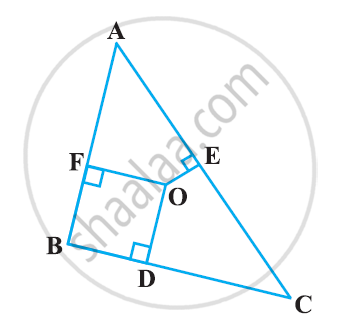(i) OA2 + OB2 + OC2 − OD2 − OE2 − OF2 = AF2 + BD2 + CE2

(ii) AF2 + BD2 + CE= AE2 + CD2 + BF2

Ex. 6.5 | Q 9 | Page 151

A ladder 10 m long reaches a window 8 m above the ground. Find the distance of the foot of the ladder from base of the wall.

Ex. 6.5 | Q 10 | Page 151

A guy wire attached to a vertical pole of height 18 m is 24 m long and has a stake attached to the other end. How far from the base of the pole should the stake be driven so that the wire will be taut?

Ex. 6.5 | Q 11 | Page 151

An aeroplane leaves an airport and flies due north at a speed of 1,000 km per hour. At the same time, another aeroplane leaves the same airport and flies due west at a speed of 1,200 km per hour. How far apart will be the two planes after 1 1/2 hours?

Ex. 6.5 | Q 12 | Page 151

Two poles of heights 6 m and 11 m stand on a plane ground. If the distance between the feet of the poles is 12 m, find the distance between their tops.

Ex. 6.5 | Q 13 | Page 151

D and E are points on the sides CA and CB respectively of a triangle ABC right angled at C. Prove that AE+ BD2 = AB2 + DE2

Ex. 6.5 | Q 14 | Page 151

The perpendicular from A on side BC of a Δ ABC intersects BC at D such that DB = 3CD . Prove that 2AB2 = 2AC2 + BC2.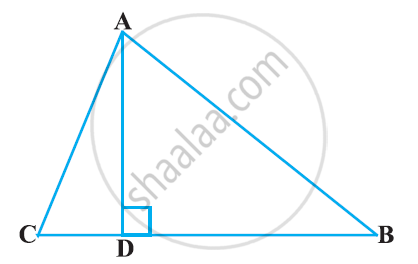Ex. 6.5 | Q 15 | Page 151

In an equilateral triangle ABC, D is a point on side BC such that BD = 1/3BC . Prove that 9 AD2 = 7 AB2

Ex. 6.5 | Q 16 | Page 151

In an equilateral triangle, prove that three times the square of one side is equal to four times the square of one of its altitudes.

Ex. 6.5 | Q 17 | Page 151

Tick the correct answer and justify: In ΔABC, AB = 6sqrt3 cm, AC = 12 cm and BC = 6 cm.

The angle B is:

• 120°

• 60°

• 90°

• 45°

#### NCERT solutions for Class 10 Mathematics Textbook Chapter 6 Triangles Exercise 6.6 [Pages 152 - 153]

Ex. 6.6 | Q 1 | Page 152

In the given figure, PS is the bisector of ∠QPR of ΔPQR. Prove that (QS)/(SR) = (PQ)/(PR)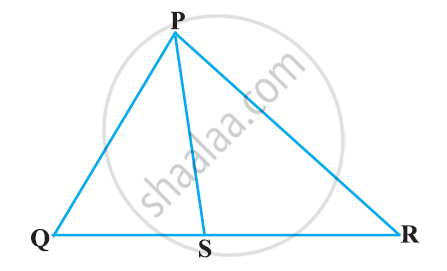Q 2 | Page 152

In the given figure, D is a point on hypotenuse AC of ΔABC, DM ⊥ BC and DN ⊥ AB, Prove that:

(i) DM2 = DN.MC

(ii) DN2 = DM.AN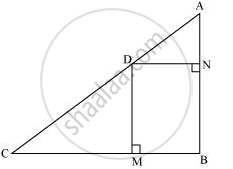Ex. 6.6 | Q 3 | Page 152

In the given figure, ABC is a triangle in which ∠ABC> 90° and AD ⊥ CB produced. Prove that AC2 = AB2 + BC2 + 2BC.BD.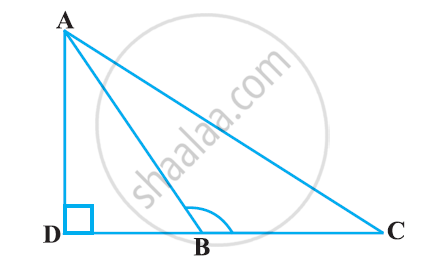Ex. 6.6 | Q 4 | Page 152

In the given figure, ABC is a triangle in which ∠ABC < 90° and AD ⊥ BC. Prove that AC2 = AB2 + BC2 − 2BC.BD.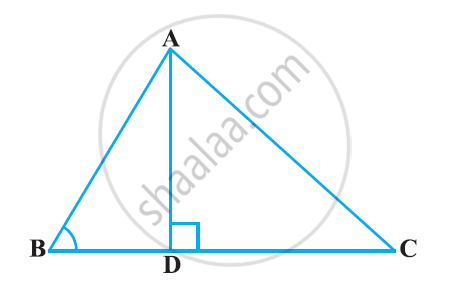Ex. 6.6 | Q 5 | Page 152

In the given figure, AD is a median of a triangle ABC and AM ⊥ BC. Prove that: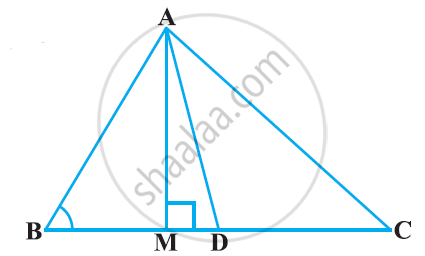1)AC^2 = AD^2 + BC . DM + ((BC)/2)^2

2) AB^2 = AD^2 – BC . DM + ((BC)/2)^2

3) AC^2 + AB^2 = 2 AD^2 + 1/2(BC)^2

Ex. 6.6 | Q 6 | Page 153

Prove that the sum of the squares of the diagonals of parallelogram is equal to the sum of the squares of its sides.

Ex. 6.6 | Q 7 | Page 153

In the given figure, two chords AB and CD intersect each other at the point P. prove that:

(i) ΔAPC ∼ ΔDPB

(ii) AP.BP = CP.DP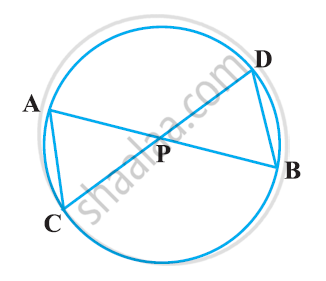Ex. 6.6 | Q 8 | Page 153

In the given figure, two chords AB and CD of a circle intersect each other at the point P (when produced) outside the circle. Prove that

(i) ΔPAC ∼ ΔPDB

(ii) PA.PB = PC.PD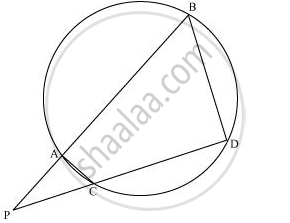Ex. 6.6 | Q 9 | Page 153

In the given figure, D is a point on side BC of ΔABC such that ∠ADC=∠BAC . Prove that AD is the bisector of ∠BAC.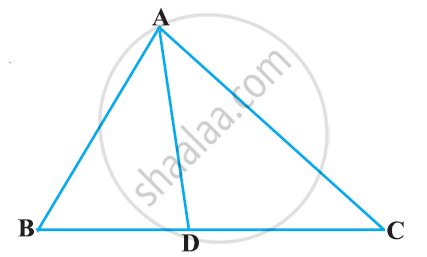Ex. 6.6 | Q 10 | Page 153

Nazima is fly fishing in a stream. The tip of her fishing rod is 1.8 m above the surface of the water and the fly at the end of the string rests on the water 3.6 m away and 2.4 m from a point directly under the tip of the rod. Assuming that her string (from the tip of her rod to the fly) is taut, ho much string does she have out (see Figure)? If she pulls in the string at the rate of 5 cm per second, what will be the horizontal distance of the fly from her after 12 seconds?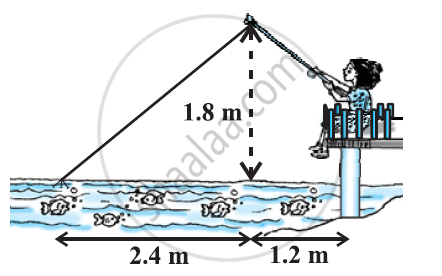## Chapter 6: Triangles

Ex. 6.1Ex. 6.2Ex. 6.3Ex. 6.4Ex. 6.5Ex. 6.6Others## NCERT solutions for Class 10 Mathematics Textbook chapter 6 - Triangles

NCERT solutions for Class 10 Mathematics Textbook chapter 6 (Triangles) include all questions with solution and detail explanation. This will clear students doubts about any question and improve application skills while preparing for board exams. The detailed, step-by-step solutions will help you understand the concepts better and clear your confusions, if any. Shaalaa.com has the CBSE Class 10 Mathematics Textbook solutions in a manner that help students grasp basic concepts better and faster.

Further, we at Shaalaa.com provide such solutions so that students can prepare for written exams. NCERT textbook solutions can be a core help for self-study and acts as a perfect self-help guidance for students.

Concepts covered in Class 10 Mathematics Textbook chapter 6 Triangles are Similar Figures, Similarity of Triangles, Basic Proportionality Theorem Or Thales Theorem, Criteria for Similarity of Triangles, Areas of Similar Triangles, Pythagoras Theorem, Similarity Triangle Theorem, Application of Pythagoras Theorem in Acute Angle and Obtuse Angle, Triangles Examples and Solutions, Angle Bisector, Similarity Examples and Solutions, Ratio of Sides of Triangle, Similar Figures, Similarity of Triangles, Basic Proportionality Theorem Or Thales Theorem, Criteria for Similarity of Triangles, Areas of Similar Triangles, Pythagoras Theorem, Similarity Triangle Theorem, Application of Pythagoras Theorem in Acute Angle and Obtuse Angle, Triangles Examples and Solutions, Angle Bisector, Similarity Examples and Solutions, Ratio of Sides of Triangle.

Using NCERT Class 10 solutions Triangles exercise by students are an easy way to prepare for the exams, as they involve solutions arranged chapter-wise also page wise. The questions involved in NCERT Solutions are important questions that can be asked in the final exam. Maximum students of CBSE Class 10 prefer NCERT Textbook Solutions to score more in exam.

Get the free view of chapter 6 Triangles Class 10 extra questions for Class 10 Mathematics Textbook and can use Shaalaa.com to keep it handy for your exam preparation

S